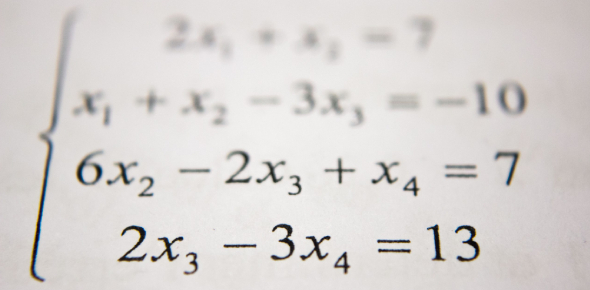# Math Quiz For Grade 7

10 Questions | Attempts: 128300
ShareSettingsAre you ready to test your math knowledge with math quiz for grade 7? Mathematics is undoubtedly one of the most interesting subjects and when understood properly, it becomes the most scoring subject as well! To test your knowledge, we've designed here a short math quiz for the students of grade 7. It will cover questions from topics such as decimals, fractions, angle measures, percent, etc. So, take this quiz and find out if you are a real math scholar or not!

• 1.
Double bar graphs are used to...
• A.

Give a detailed view of the information

• B.

Show similarities among the information.

• C.

Compare the information

• D.

None of these

• 2.
The percentage can be out of any number.
• A.

True

• B.

False

• 3.
How many degrees are there in a circle?
• A.

100

• B.

360

• C.

10

• D.

650

• 4.
What do we use to display information in circle graphs?
• A.

Percent

• B.

Degrees

• C.

Fraction

• D.

Angles

• 5.
You can have decimal oneths.
• A.

True

• B.

False

• 6.
Which would you use if you were to display the average snowfall in Montreal over a time period of one week?
• A.

Bar Graph

• B.

Double Bar Graph

• C.

Double Line Graph

• D.

None of these

• 7.
Tally charts are grouped in 5's.
• A.

True

• B.

False

• 8.
What is the formula for calculating the area of a square?
• A.

Side*side

• B.

4*side

• C.

• D.

• 9.
Numerators are over the denominator.
• A.

True

• B.

False

• 10.
25% is [Blank]__ as a fraction, when reduced.
• A.

1/1

• B.

1/2

• C.

1/3

• D.

1/4

## Related TopicsBack to top
×

Wait!
Here's an interesting quiz for you.